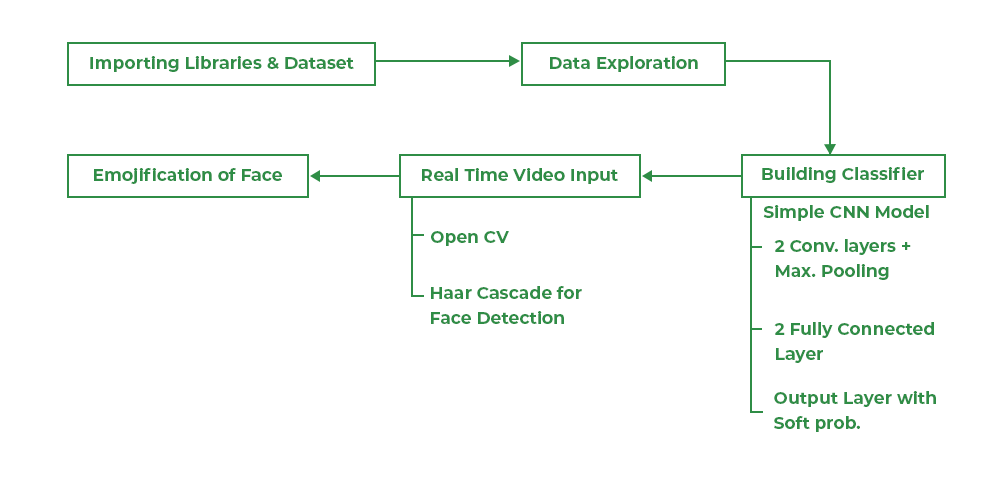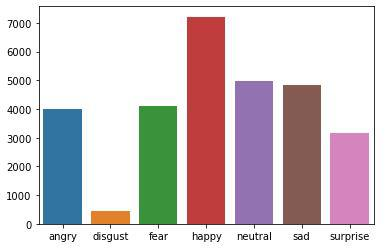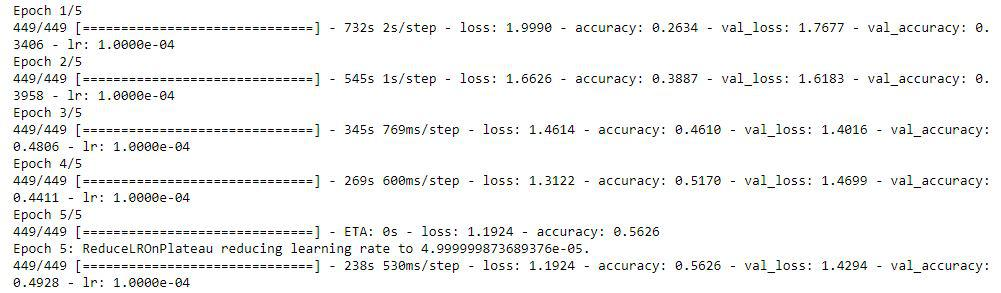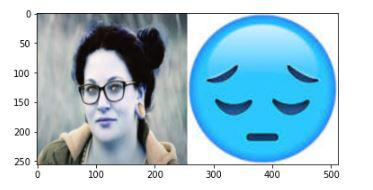# Emojify using Face Recognition with Machine Learning

In this article, we will learn how to implement a modification app that will show an emoji of expression which resembles the expression on your face. This is a fun project based on computer vision in which we use an image classification model in reality to classify different expressions of a person.

This project will be implemented in two parts:

1. Building an image classification model which can classify facial images with different expressions on them.
2. Extracting the face from an image and then classifying the expression on it using the classifier.Data flow of the project

## Modules Used

Python libraries make it very easy for us to handle the data and perform typical and complex tasks with a single line of code.

• Pandas This library helps to load the data frame in a 2D array format and has multiple functions to perform analysis tasks in one go.
• Numpy Numpy arrays are very fast and can perform large computations in a very short time.
• Matplotlib This library is used to draw visualizations.
• Sklearn – This module contains multiple libraries having pre-implemented functions to perform tasks from data preprocessing to model development and evaluation.
• OpenCVThis is an open-source library mainly focused on image processing and handling.
• Tensorflow – This is an open-source library that is used for Machine Learning and Artificial intelligence and provides a range of functions to achieve complex functionalities with single lines of code.

## Python3

 `import` `numpy as np ` `import` `matplotlib.pyplot as plt ` `import` `seaborn as sb ` `from` `PIL ``import` `Image ` ` `  `from` `sklearn ``import` `metrics ` ` `  `import` `cv2 ` `import` `os ` ` `  `from` `glob ``import` `glob ` ` `  `import` `tensorflow as tf ` `from` `tensorflow ``import` `keras ` `from` `keras ``import` `layers ` `from` `keras.preprocessing.image ``import` `ImageDataGenerator ` ` `  `import` `warnings ` `warnings.filterwarnings(``'ignore'``)`

## Importing Dataset

The dataset which we will use contains around 30,000 images for seven different categories of emotions. Although the images are of very small size (48, 48) RGB format images. As the images are way too small for real-life applications. Also, our model will face difficulty in learning the patterns of different expressions on human faces.

## Python3

 `# Extracting the compressed dataset. ` `from` `zipfile ``import` `ZipFile ` `data_path ``=` `'fer2013.zip'` ` `  `with ZipFile(data_path,``'r'``) as ``zip``: ` `  ``zip``.extractall() ` `  ``print``(``'The data set has been extracted.'``)`

`The data set has been extracted.`

## Data Exploration

In this section, we will try to explore the data by visualizing the number of images provided for each category for the train and the test data.

## Python3

 `path ``=` `'train'` `classes ``=` `os.listdir(path) ` `classes`

Output:

`['angry', 'disgust', 'fear', 'happy', 'neutral', 'sad', 'surprise']`

These are the seven classes of emotions that we have here.

## Python3

 `count ``=` `[] ` `for` `cat ``in` `classes: ` `    ``count.append(``len``(os.listdir(f``'{path}/{cat}'``))) ` `sb.barplot(classes, count) ` `plt.show()`

Output:Countplot of images present in each category

One of the important observations here we can draw is the high data imbalance in the “disgust” category. Due to this reason maybe our model will not perform well on this class of images.

## Model Development

From this step onward we will use the TensorFlow library to build our CNN model. Keras framework of the tensor flow library contains all the functionalities that one may need to define the architecture of a Convolutional Neural Network and train it on the data.

#### Model Architecture

We will implement a Sequential model which will contain the following parts:

• Three Convolutional Layers followed by MaxPooling Layers.
• The Flatten layer to flatten the output of the convolutional layer.
• Then we will have two fully connected layers followed by the output of the flattened layer.
• We have included some BatchNormalization layers to enable stable and fast training and a Dropout layer before the final layer to avoid any possibility of overfitting.
• The final layer is the output layer which outputs soft probabilities for the seven classes.

## Python3

 `train_datagen ``=` `ImageDataGenerator(rescale``=``1.``/``255``) ` `val_datagen ``=` `ImageDataGenerator(rescale``=``1.``/``255``) ` ` `  `train_gen ``=` `train_datagen.flow_from_directory( ` `        ``'train'``, ` `        ``target_size``=``(``48``,``48``), ` `        ``batch_size``=``64``, ` `        ``color_mode``=``"grayscale"``, ` `        ``class_mode``=``'categorical'``) ` ` `  `val_gen ``=` `val_datagen.flow_from_directory( ` `        ``'test'``, ` `        ``target_size``=``(``48``,``48``), ` `        ``batch_size``=``64``, ` `        ``color_mode``=``"grayscale"``, ` `        ``class_mode``=``'categorical'``)`

Output:

```Found 28709 images belonging to 7 classes.
Found 7178 images belonging to 7 classes.```

Let’s store the labels and which are assigned to different classes of the emotions.

## Python3

 `emotions ``=` `list``(train_gen.class_indices.keys())`

Let’s define the model’s architecture.

## Python3

 `model ``=` `keras.models.Sequential([ ` `                                    ``layers.Conv2D(``32``,(``3``,``3``),activation``=``'relu'``,input_shape``=``(``48``, ``48``, ``1``)),                                     ` `                                    ``layers.Conv2D(``64``,(``3``,``3``),activation``=``'relu'``), ` `                                    ``layers.MaxPooling2D(``2``,``2``), ` `                                  `  `                                     `  `                                    ``layers.Flatten(), ` `                                    ``layers.Dense(``64``,activation``=``'relu'``), ` `                                    ``layers.BatchNormalization(),                                 ` `                                    ``layers.Dense(``32``,activation``=``'relu'``), ` `                                    ``layers.Dropout(``0.3``), ` `                                    ``layers.BatchNormalization(), ` `                                    ``layers.Dense(``7``, activation``=``'softmax'``) ` `])`

While compiling a model we provide these three essential parameters:

• optimizer – This is the method that helps to optimize the cost function by using gradient descent.
• loss – The loss function by which we monitor whether the model is improving with training or not.
• metrics – This helps to evaluate the model by predicting the training and the validation data.

## Python3

 `model.``compile``( ` `    ``optimizer ``=` `'adam'``, ` `    ``loss ``=` `'categorical_crossentropy'``, ` `    ``metrics``=``[``'accuracy'``] ` `)`

### Callback

Callbacks are used to check whether the model is improving with each epoch or not. If not then what are the necessary steps to be taken like ReduceLROnPlateau decreases learning rate further. Even then if model performance is not improving then training will be stopped by EarlyStopping. We can also define some custom callbacks to stop training in between if the desired results have been obtained early.

## Python3

 `from` `keras.callbacks ``import` `EarlyStopping, ReduceLROnPlateau ` ` `  `class` `myCallback(tf.keras.callbacks.Callback): ` `  ``def` `on_epoch_end(``self``, epoch, logs``=``{}): ` `    ``if` `logs.get(``'val_accuracy'``) > ``0.90``: ` `      ``print``(``'\n Validation accuracy has reached upto 90% so, stopping further training.'``) ` `      ``self``.model.stop_training ``=` `True` ` `  `es ``=` `EarlyStopping(patience``=``3``, monitor``=``'val_accuracy'``, restore_best_weights``=``True``) ` `lr ``=` `ReduceLROnPlateau(monitor ``=` `'val_loss'``, patience ``=` `2``, factor``=``0.5``, verbose``=``1``)`

Now we will train our model:

## Python3

 `history ``=` `model.fit(train_gen, ` `                    ``validation_data ``=` `val_gen, ` `                    ``epochs ``=` `5``, ` `                    ``verbose ``=` `1``, ` `                    ``callbacks ``=` `[es, lr, myCallback()])`

Output:Training progress of the model

The first part of our project is to build a classifier that can classify different emotions.

## Predicting Emoji in Real Time

This is part two of this project where we will predict expressions on a person’s face and show an emoji based on that in real-time. To detect the face in a video feed we will use haar_cascade_classifier.

Below is a helper function that will be used to plot the images.

## Python3

 `def` `plot_image(img, emoj): ` `    ``wmin ``=` `256` `    ``hmin ``=` `256` ` `  `    ``emoj ``=` `cv2.resize(emoj, (wmin, hmin)) ` `    ``img ``=` `cv2.resize(img, (wmin, hmin)) ` `    ``cv2.imshow(``'Images'``, cv2.hconcat([img, emoj]))`

Now we will capture the video and by using the haar_cascade_classifier we will predict the emoji for that expression.

## Python3

 `face_cascade ``=` `cv2.CascadeClassifier(``'haarcascade_frontalface_default.xml'``) ` ` `  `img ``=` `cv2.imread(``'sad.jpg'``) ` `gray ``=` `cv2.cvtColor(img, cv2.COLOR_BGR2GRAY) ` `faces ``=` `face_cascade.detectMultiScale(gray) ` ` `  `for` `(x, y, w, h) ``in` `faces: ` `    ``gray ``=` `cv2.resize(gray[x:x``+``w``-``10``,y:y``+``h``+``10``], (``48``,``48``)) ` `    ``gray ``=` `np.expand_dims(gray, axis``=``-``1``) ` `    ``gray ``=` `np.expand_dims(gray, axis``=``0``) ` ` `  `    ``pred ``=` `model.predict(gray) ` `    ``idx ``=` `pred.argmax(axis``=``-``1``)[``0``] ` ` `  `    ``emoj ``=` `cv2.imread(f``'emojis/{classes[idx]}.jpg'``) ` ` `  `    ``plot_image(img, emoj) ` ` `  `    ``if` `cv2.waitKey(``1``) & ``0xFF` `=``=` `ord``(``'q'``): ` `        ``break` `     `  `cv2.destroyAllWindows()`

Output:Image along with the predicted emoji

Here is code for video:

## Python3

 `face_cascade ``=` `cv2.CascadeClassifier(``'haarcascade_frontalface_default.xml'``) ` ` `  `cap ``=` `cv2.VideoCapture(``0``) ` ` `  ` `  `while``(``True``): ` `    ``ret, img ``=` `cap.read() ` `    ``img ``=` `cv2.resize(img, (``256``, ``256``)) ` `    ``gray ``=` `cv2.cvtColor(img, cv2.COLOR_BGR2GRAY) ` `    ``faces ``=` `face_cascade.detectMultiScale(gray) ` ` `  `    ``if` `len``(faces)>``0``: ` `        ``for` `(x, y, w, h) ``in` `faces: ` `            ``try``: ` `                ``gray ``=` `cv2.resize(gray[x:x``+``w,y:y``+``h], (``48``,``48``)) ` `            ``except``: ` `                ``break` `             `  `            ``gray ``=` `np.expand_dims(gray, axis``=``-``1``) ` `            ``gray ``=` `np.expand_dims(gray, axis``=``0``) ` ` `  `            ``pred ``=` `model.predict(gray) ` `            ``idx ``=` `pred.argmax(axis``=``-``1``)[``0``] ` ` `  `            ``emoj ``=` `cv2.imread(f``'emojis/{emotions[idx]}.jpg'``) ` ` `  `            ``plot_image(img, emoj) ` `    ``else``: ` `        ``emoj ``=` `cv2.imread(``'NofaceDetected.jpeg'``) ` `        ``plot_image(img, emoj) ` ` `  `    ``if` `cv2.waitKey(``10``) & ``0xFF` `=``=` `ord``(``'q'``): ` `        ``break` `     `  `cap.release() ` `cv2.destroyAllWindows()`

#### Conclusion:

There might be some discrepancies in the result because the classifier we build can predict emotions with only 55% accuracy. If we will use a better dataset in terms of size for example then the accuracy of the model increase and so does the emoji predicted by the model. The above shown is a use case of the classifier for a static image. Just replace the last code block with the below one and you’ll be able to predict the emoji in real time for your images.

Whether you're preparing for your first job interview or aiming to upskill in this ever-evolving tech landscape, GeeksforGeeks Courses are your key to success. We provide top-quality content at affordable prices, all geared towards accelerating your growth in a time-bound manner. Join the millions we've already empowered, and we're here to do the same for you. Don't miss out - check it out now!